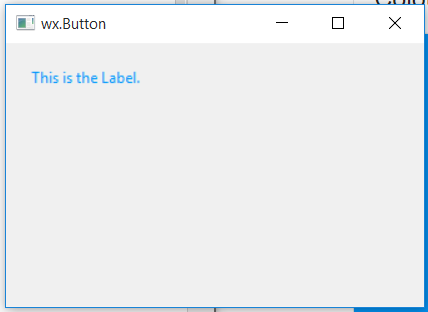# wxPython – SetForegroundColour() function in wx.StaticText

In this article we are going to learn about SetForegroundColour() function associated with wx.StaticText class of wxPython. SetForegroundColour() function is simply used to set foreground colour of a static text to a different colour.

It takes wx.Colour argument to set the background colour.

Syntax: wx.StaticText.SetForegroundColour(self, colour)

Parameters:

Parameter Input Type Description
colour wx.Colour Foreground colour to set.

Code Example:

 `import` `wx ` ` `  ` `  `class` `Example(wx.Frame): ` ` `  `    ``def` `__init__(``self``, ``*``args, ``*``*``kwargs): ` `        ``super``(Example, ``self``).__init__(``*``args, ``*``*``kwargs) ` `        ``self``.InitUI() ` ` `  `    ``def` `InitUI(``self``): ` `        ``self``.locale ``=` `wx.Locale(wx.LANGUAGE_ENGLISH) ` `        ``self``.pnl ``=` `wx.Panel(``self``) ` ` `  `        ``bmp ``=` `wx.Bitmap(``'right.png'``) ` `        ``# CREATE STATICTEXT AT POINT (20, 20) ` `        ``self``.st ``=` `wx.StaticText(``self``.pnl, ``id` `=` `1``, label ``=``"This is the Label."``,  ` `                                ``pos ``=``(``20``, ``20``), size ``=` `wx.DefaultSize,  ` `                           ``style ``=` `wx.ST_ELLIPSIZE_MIDDLE, name ``=``"statictext"``) ` ` `  `        ``# SET FOREGROUND COLOUR TO YELLOW ` `        ``self``.st.SetForegroundColour((``3``, ``152``, ``252``, ``255``)) ` `        ``self``.SetSize((``350``, ``250``)) ` `        ``self``.SetTitle(``'wx.Button'``) ` `        ``self``.Centre() ` ` `  `def` `main(): ` `    ``app ``=` `wx.App() ` `    ``ex ``=` `Example(``None``) ` `    ``ex.Show() ` `    ``app.MainLoop() ` ` `  ` `  `if` `__name__ ``=``=` `'__main__'``: ` `    ``main() `

Output Window:Whether you're preparing for your first job interview or aiming to upskill in this ever-evolving tech landscape, GeeksforGeeks Courses are your key to success. We provide top-quality content at affordable prices, all geared towards accelerating your growth in a time-bound manner. Join the millions we've already empowered, and we're here to do the same for you. Don't miss out - check it out now!

Previous
Next